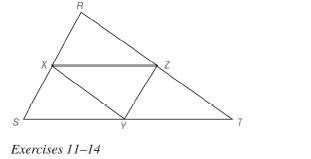Chapter 4.2, Problem 11EElementary Geometry For College St...

7th Edition
Alexander + 2 others
ISBN: 9781337614085

Solutions

Chapter
SectionElementary Geometry For College St...

7th Edition
Alexander + 2 others
ISBN: 9781337614085
Textbook Problem

In Exercises 11 to 14 , assume that X , Y , and Z are midpoints of the sides of ∆ R S T .If R S = 12 , S T = 14 , and R T = 16 , find: a) X Y b) X Z c) Y ZTo determine

a)

To find:

The value of XY

Explanation

Approach:

The line segment that joins the midpoints of two sides of a triangle is parallel to the third side and has a length equal to one half the length of the third side.

Calculation:

Given,

X, Y, and Z are the midpoints.

RS=12,

ST=14, and

RT=16

Here, X and Y are the midpoints of the sides RS and ST of the triangle RST

To determine

b)

To find:

The value of XZ.

To determine

c)

To find:

The value of YZ.

Still sussing out bartleby?

Check out a sample textbook solution.

See a sample solution

The Solution to Your Study Problems

Bartleby provides explanations to thousands of textbook problems written by our experts, many with advanced degrees!

Get Started

Which of the following three graphs is the graph of ? Explain your choice.

Mathematical Applications for the Management, Life, and Social Sciences

Show that the function f(x)={x4sin(1/x)ifx00ifx=0 is continuous on ( , ).

Single Variable Calculus: Early Transcendentals, Volume I

In Exercises 99-106, factor out the greatest common factor from each expression. 105. 2x5/232x3/2

Applied Calculus for the Managerial, Life, and Social Sciences: A Brief Approach

Find numbers a and b such that limx0ax+b2x=1.

Single Variable Calculus: Early Transcendentals

Write the following numbers in word form. .00938

Contemporary Mathematics for Business & Consumers

Prove the Cauchy-Schwarz inequality |u.v|uv.

Calculus: Early Transcendental Functions (MindTap Course List)

Find for y defined implicity by .

Study Guide for Stewart's Multivariable Calculus, 8th